```final gds oasis
```
2 files changed

# General Purpose Bandgap Reference Circuit - avsdbgp_3v3_sky130_v2

A Bandgap Reference is an analog circuit, which is used to provide a constant output reference voltage being independent of Temperature, Process and Supply voltage variations. The analog IP avsdbgp_3v3 is a General Purpose Bandgap Reference ciruit, which is designed using Sky130nm technology in this project.

For detailed information regarding the Bandgap Reference circuit click here. The Design specifications provided for avsdbgp_3v3 can be found here.

• Performance parameters Bandgap Reference IP

• Circuit Implementation of Bandgap Reference IP

• Block Diagram of Bandgap Reference IP
• Schematic of Bandgap Reference IP

• Pre-Layout Simulation of Bandgap Reference IP circuit using Ngspice

• Ngspice
• Pre-Layout Simulation plots of Bandgap Reference IP circuit
• Vbgp v/s Temperature [ -40C - 140C] @ RL = 100M ohms plot
• Vbgp v/s VDD [ 2V - 4V] @ RL = 100M ohms plot
• Temperature Coefficient of Vbgp v/s Temperature [ -40C - 140C] @ RL = 100M ohms plot
• Voltage Coefficient of Vbgp v/s VDD [ 2V - 4V] @ RL = 100M ohms plot
• Start-Up Time of Vbgp @ RL = 100M ohms plot
• On-Off-Current of Vbgp wrt Enable @ RL = 100M ohms plot
• BGR Layout

• Magic
• Layout
• Post-Layout Simulation of Bandgap Reference IP circuit using Ngspice

• Vbgp v/s Temperature [ -40C - 140C] @ RL = 100M ohms plot
• Vbgp v/s VDD [ 2V - 4V] @ RL = 100M ohms plot
• Temperature Coefficient of Vbgp v/s Temperature [ -40C - 140C] @ RL = 100M ohms plot
• Voltage Coefficient of Vbgp v/s VDD [ 2V - 4V] @ RL = 100M ohms plot
• Start-Up Time of Vbgp @ RL = 100M ohms plot
• On-Off-Current of Vbgp wrt Enable @ RL = 100M ohms plot

# Performance parameters of Bandgap Reference IP

ParameterDescriptionMinTypeMaxUnitCondition
VBGPOutput reference voltage1.194901.197561.19764VoltT= -40 to 140C, VDD=3.3V
VBGPOutput reference voltage1.114001.197561.21933VoltVDD=2V to VDD=4V, T=27C
VDDSupply Voltage3.3VoltT=-40C to 125C
IDDSupply Current21.9636uAEN=1
IDDSupply Current24.4pAEN=0

# Circuit Implementation of Bandgap Reference IP

## Block Diagram of Bandgap Reference IP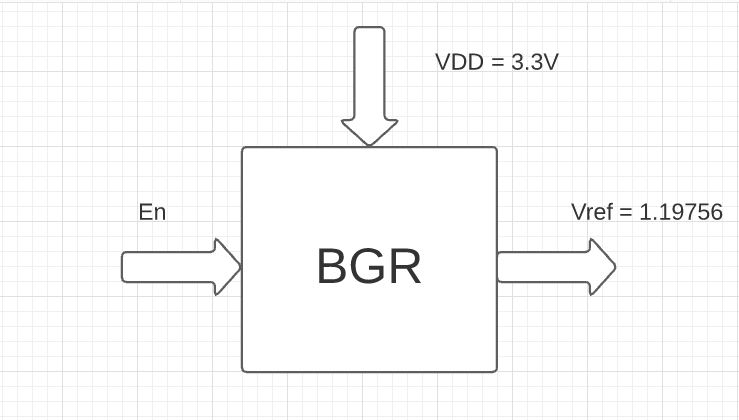## Schematic of Bandgap Reference IP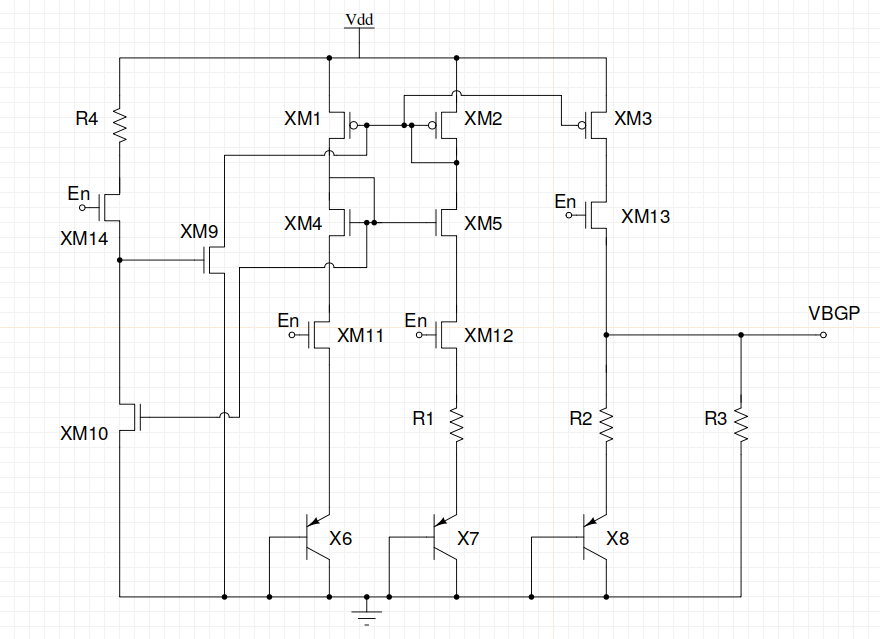The files from this repository can be downloaded and used by the following commands :-

`sudo apt install -y git`

`git clone https://github.com/vsdip/avsdbgp_3v3_sky130_v2.git`

# Pre-Layout Simulation of Bandgap Reference IP circuit

The circuit implementation of Bandgap reference IP avsdbgp_3v3 is simulated using Ngspice to analyse its performance according to the Design Specifications provided.

## Ngspice

`sudo apt-get install -y ngspice`

After successful installation, to invoke Ngspice type the following command on the terminal window.

`ngspice <circuit file to be simulated>`

## Pre- Layout Simulation plots of Bandgap Reference IP circuit

To simulate the files for Pre- Layout simulations

`cd avsdbgp_3v3_sky130_v2/Pre_Layout_Simulations/Circuits/`

## Vbgp v/s Temperature [ -40C - 140C] @ RL = 100M ohms plot

`ngspice temp_avsdbgp_3v3.cir`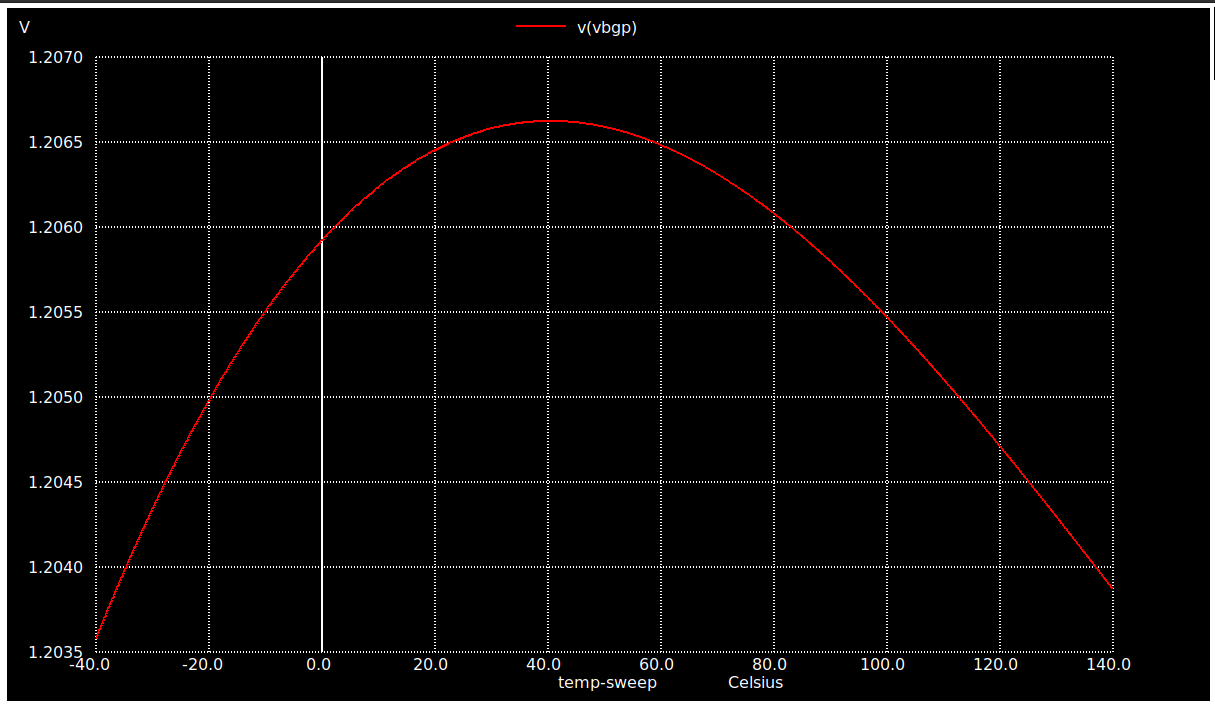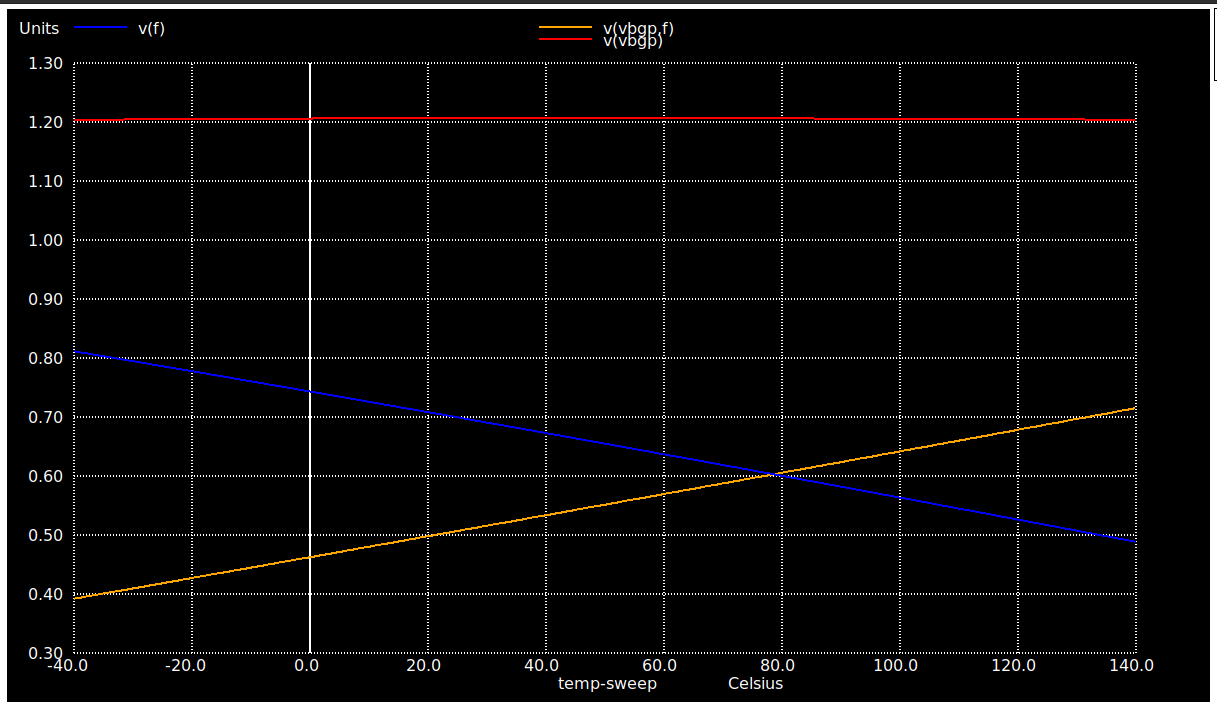## Vbgp v/s VDD [ 2V - 4V] @ RL = 100M ohms plot

`ngspice vdd_variation_avsdbgp_3v3.cir`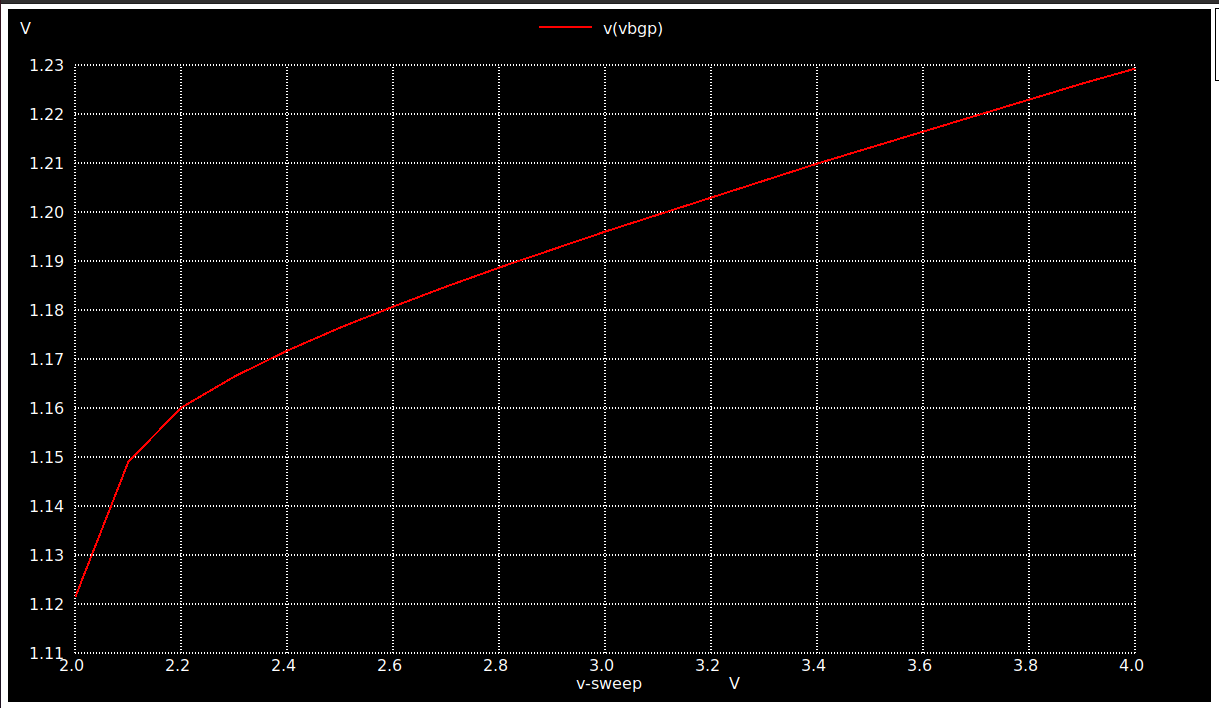## Temperature Coefficient of Vbgp v/s Temperature [ -40C - 140C] @ RL = 100M ohms plot

`ngspice Temp_coeff_avsdbgp_3v3.cir`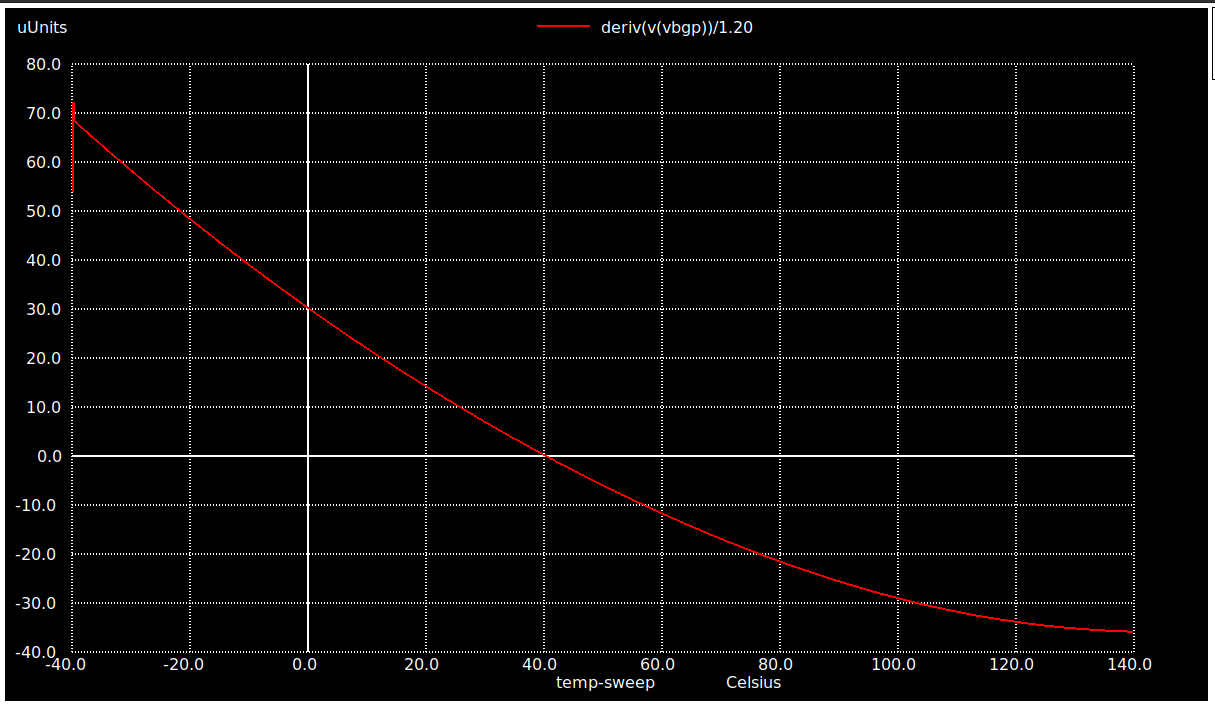## Voltage Coefficient of Vbgp v/s VDD [ 2V - 4V] @ RL = 100M ohms plot

`ngspice voltage_coeff_avsdbgp_3v3.cir`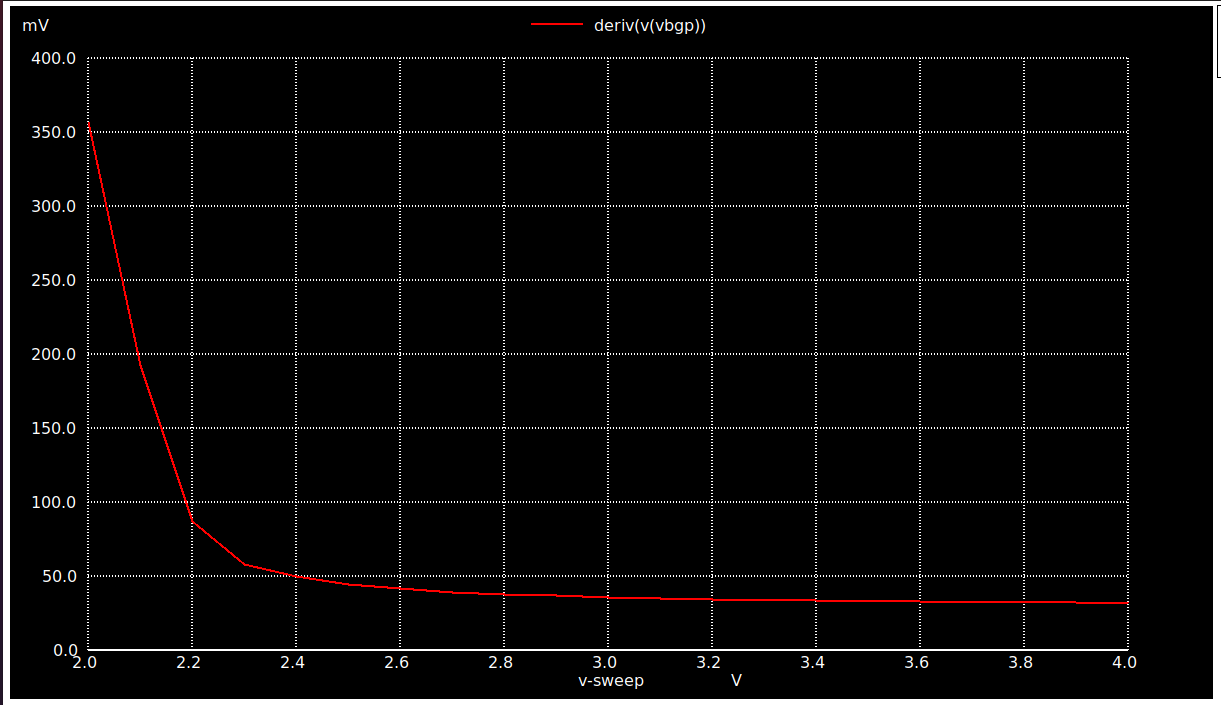## Start-Up Time of Vbgp @ RL = 100M ohms plot

`ngspice Start_up_avsdbgp_3v3.cir`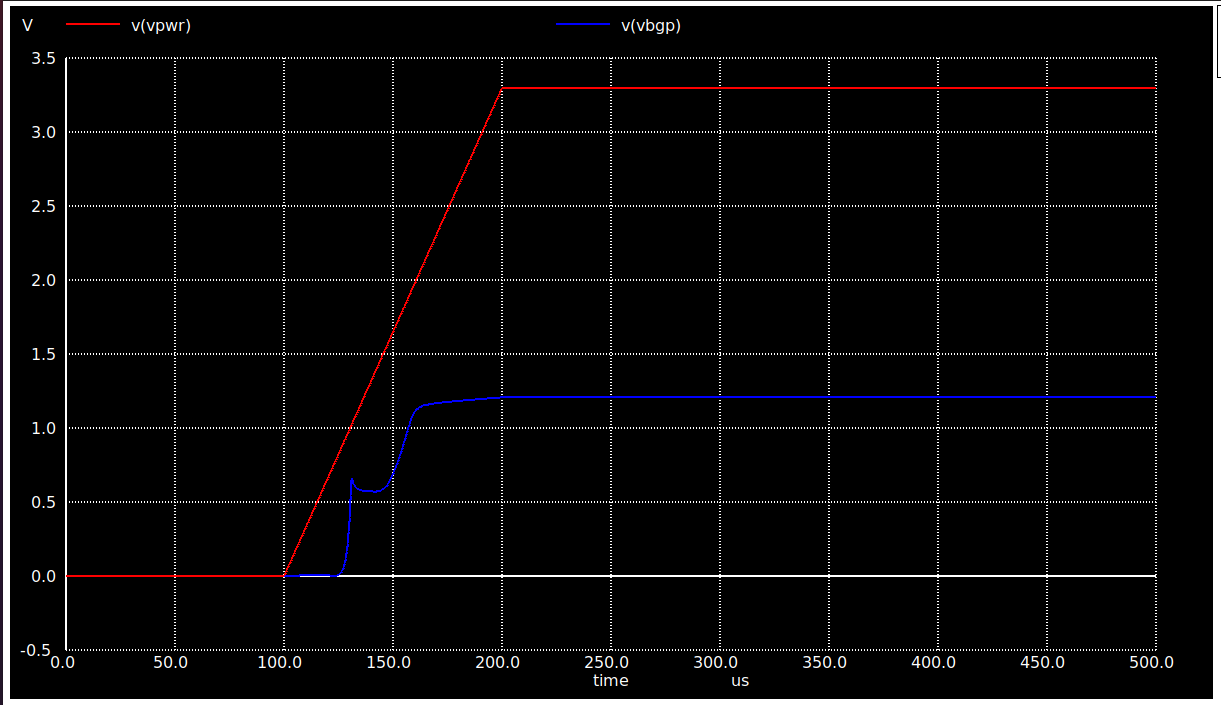## On-Off-Current of Vbgp wrt Enable @ RL = 100M ohms plot

`ngspice enable_current.cir`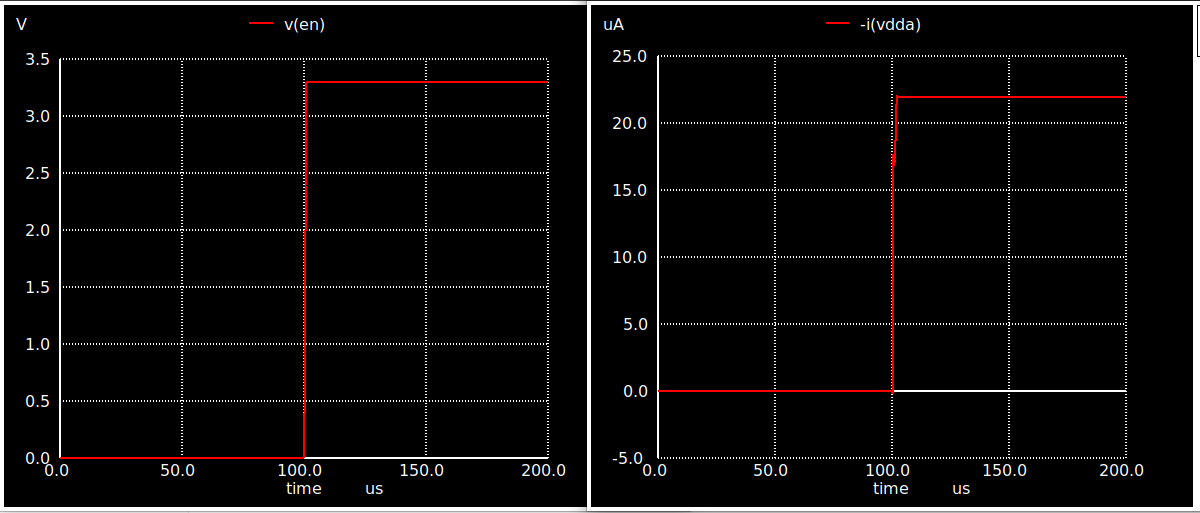# BGR Layout

## Magic

The Layout for the circuit was done using Magic Layout editor tool. To observe the layout, install magic using the following commands :-

`sudo wget "http://opencircuitdesign.com/magic/archive/magic-8.3.122.tgz" `

`tar -xvzf magic-8.3.122.tgz`

`cd magic-8.3.122`

`sudo ./configure`

`sudo make`

`sudo make install`

## Layout

After successful installation, type:-

`cd avsdbgp_3v3_sky130_v2/Layout/`

` magic -T ../libs/sky130A.tech BGR.mag`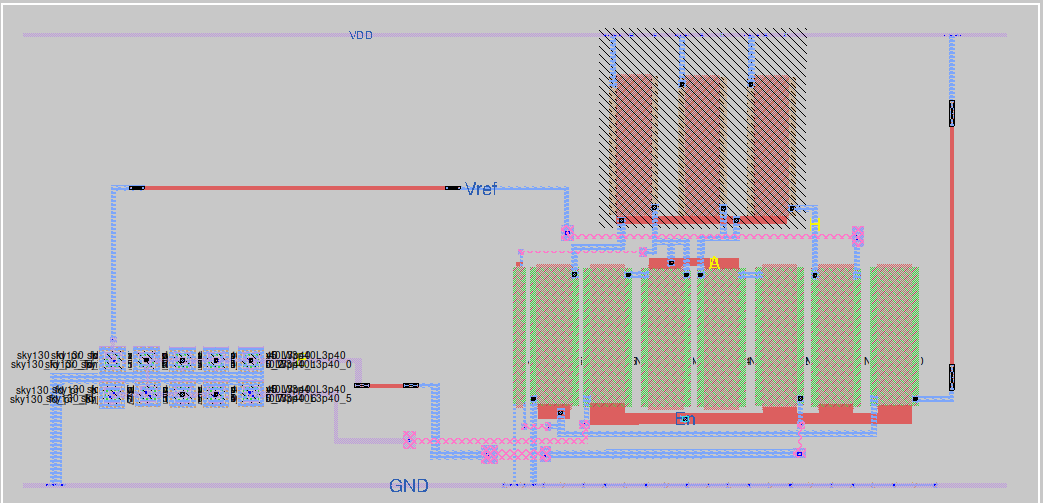# Post-Layout Simulation plots of Bandgap Reference IP circuit

To simulate the files for Post-Layout simulations using ngspice :-

`cd avsdbgp_3v3_sky130_v2/Post_Layout_Simulations/Circuits/`

## Vbgp v/s Temperature [ -40C - 140C] @ RL = 100M ohms plot

`ngspice temp_avsdbgp_3v3.spice`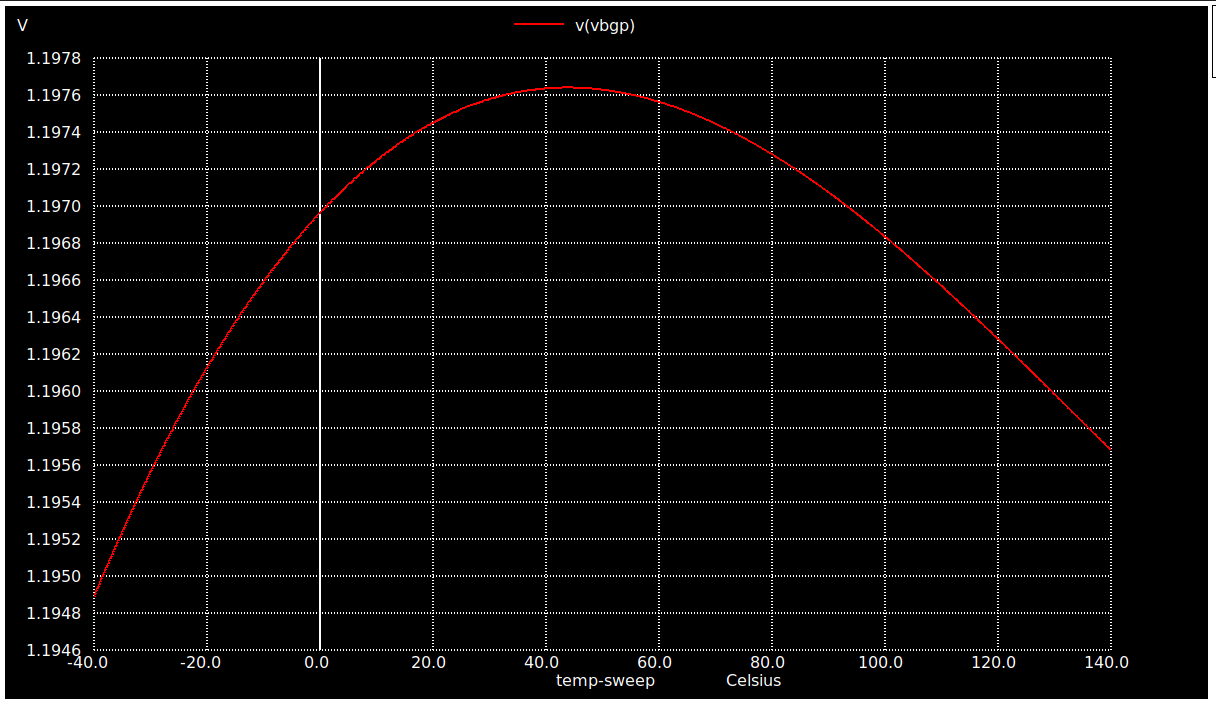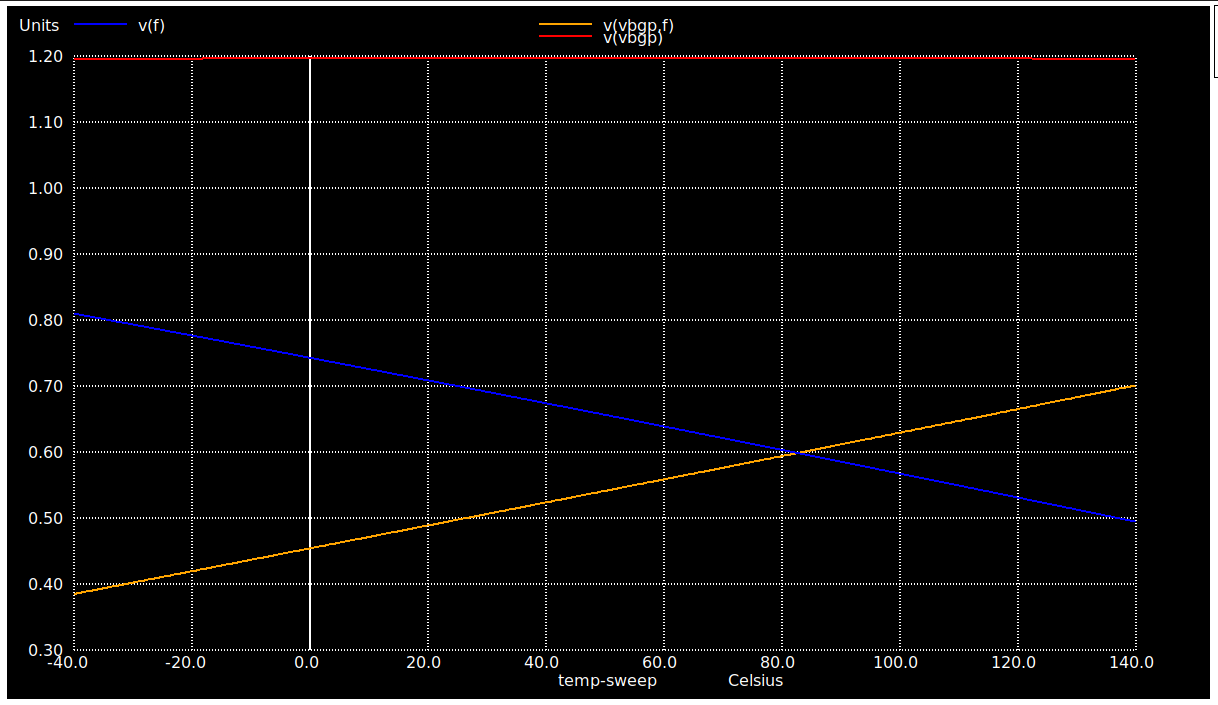## Vbgp v/s VDD [ 2V - 4V] @ RL = 100M ohms plot

`ngspice vdd_variation_avsdbgp_3v3.spice`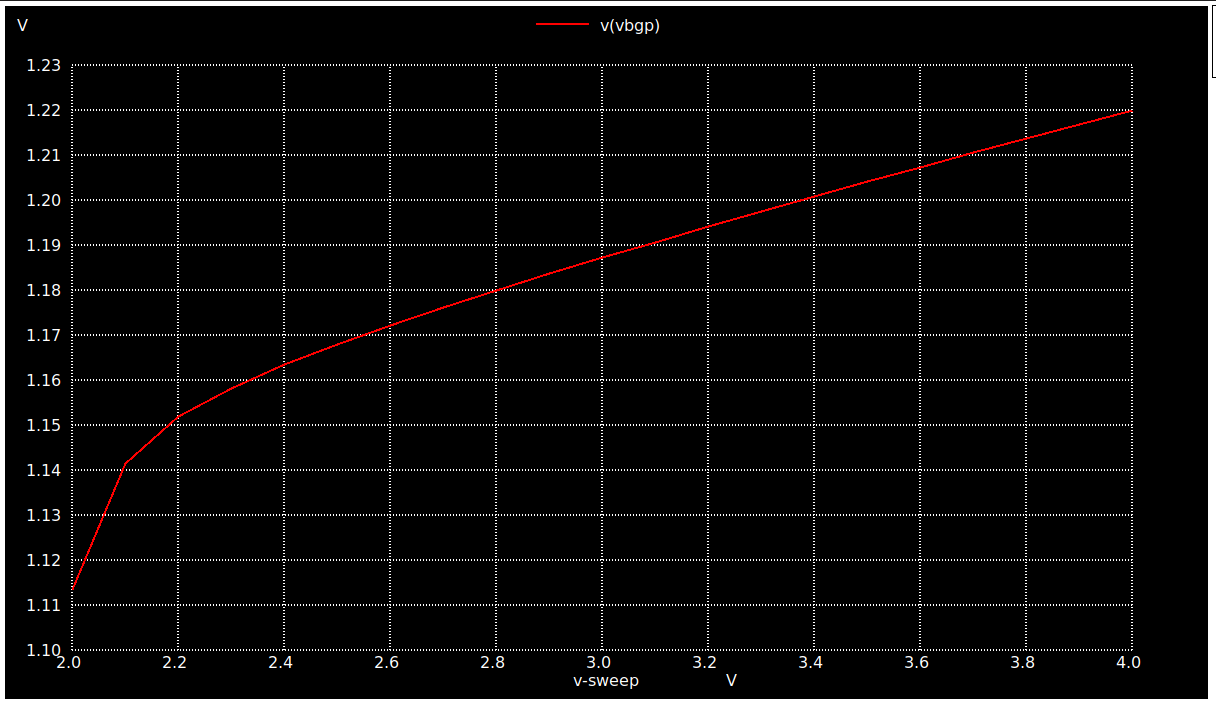## Temperature Coefficient of Vbgp v/s Temperature [ -40C - 140C] @ RL = 100M ohms plot

`ngspice Temp_coeff_avsdbgp_3v3.spice`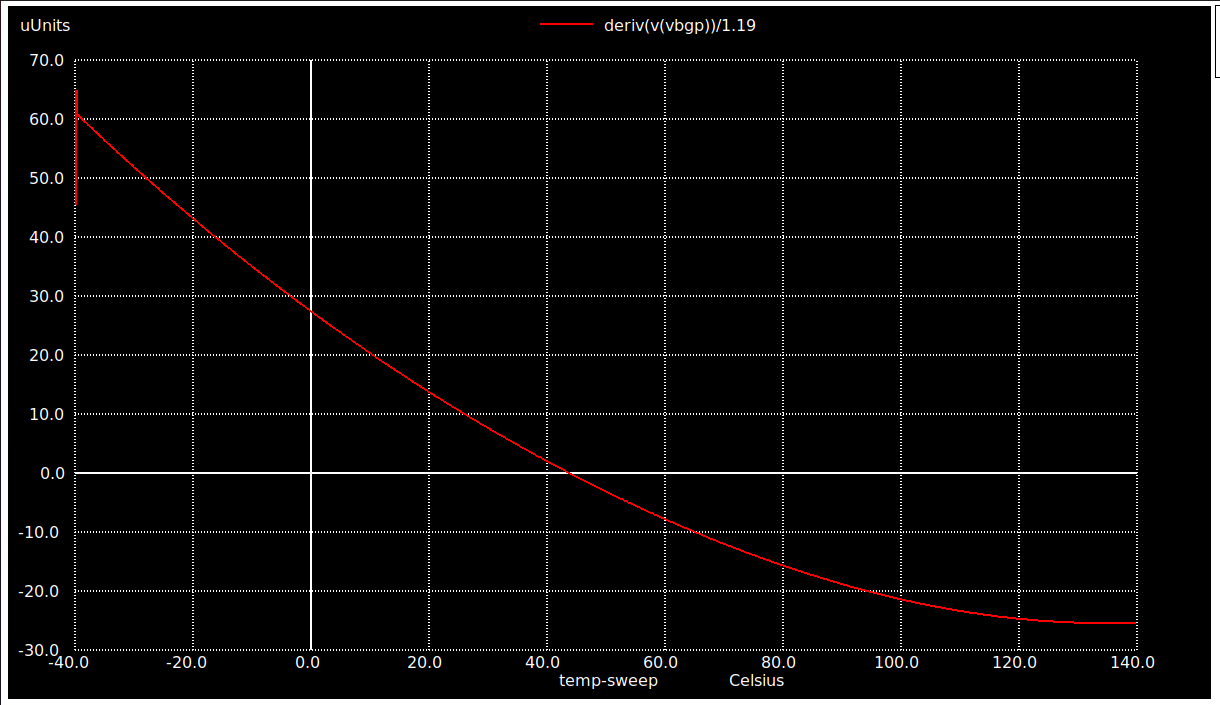## Voltage Coefficient of Vbgp v/s VDD [ 2V - 4V] @ RL = 100M ohms plot

`ngspice voltage_coeff_avsdbgp_3v3.spice`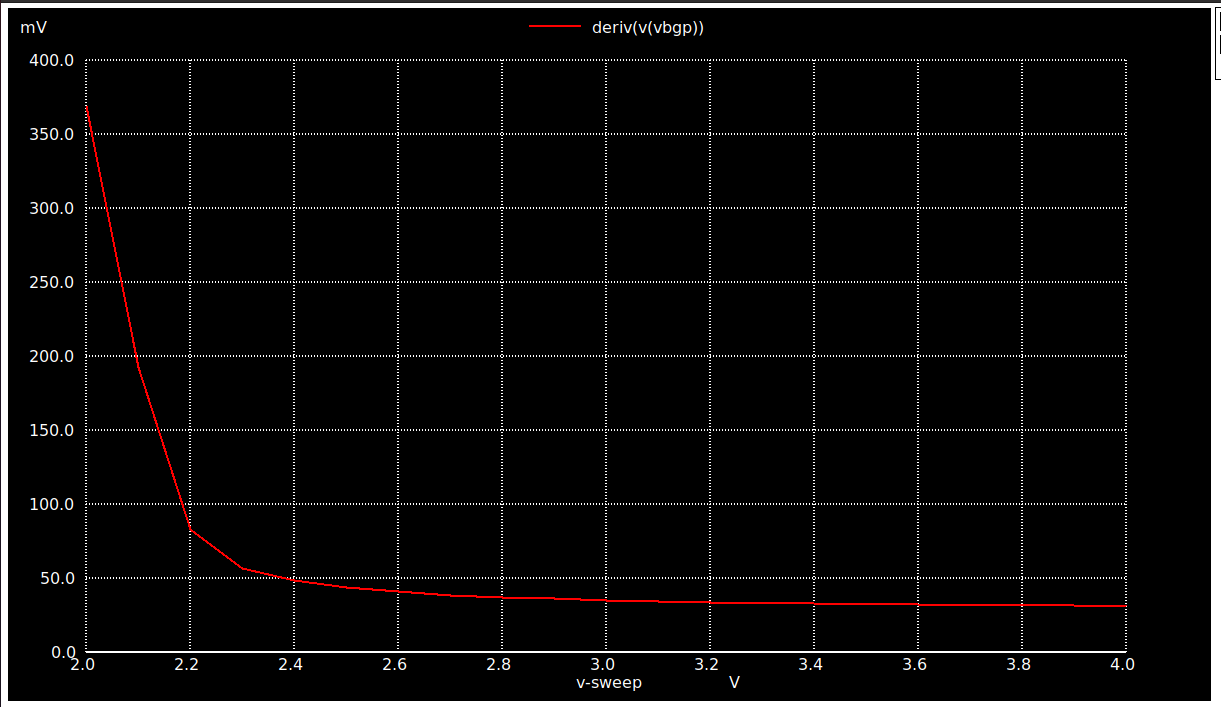## Start-Up Time of Vbgp @ RL = 100M ohms plot

`ngspice Start_up_avsdbgp_3v3.spice`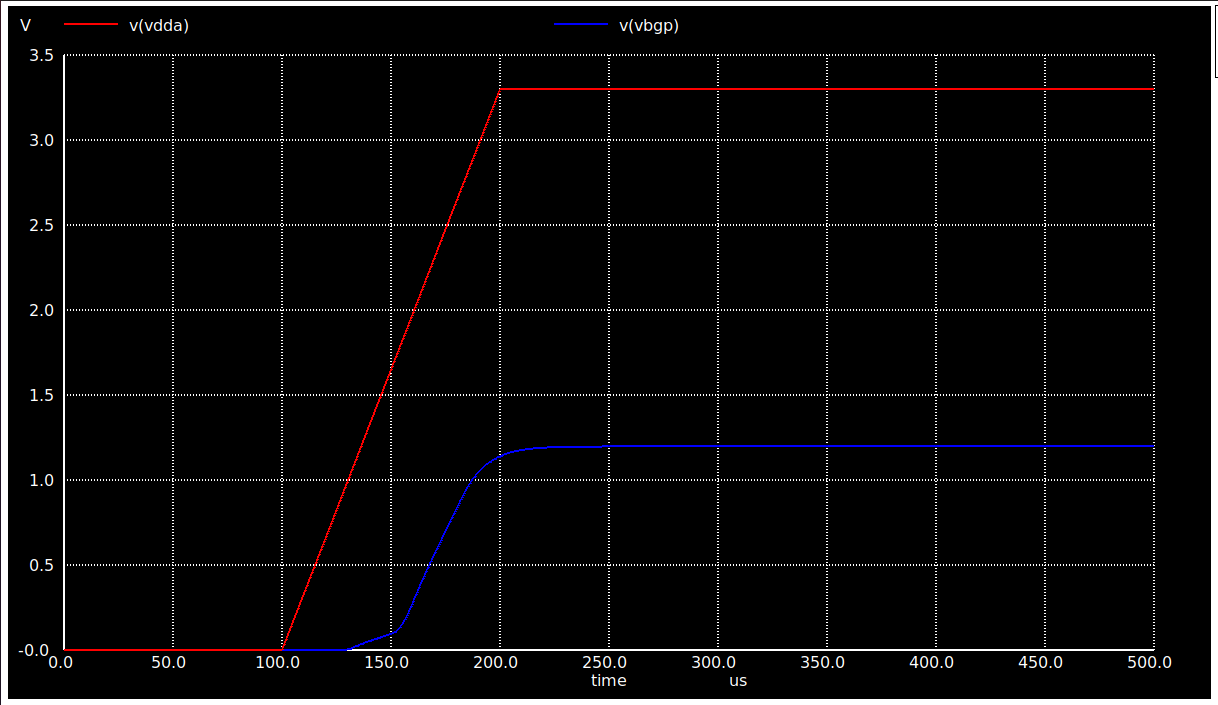# Acknowledgements

• Kunal Ghosh, Co-founder of VLSI System Design (VSD) Corp. Pvt. Ltd.
• Tim Edwards, Senior Vice President of Analog and Design at efabless corporation.
• Sheryl Corina Serrao, Undergraduate Student, Mumbai University.